Next: Example 13.4: Diverging lenses Up: Paraxial Optics Previous: Example 13.2: Convex mirrors

## Example 13.3: Converging lenses

Question: An object of height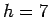cm is placed a distance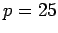cm in front of a thin converging lens of focal length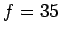cm. What is the height, location, and nature of the image? Suppose that the object is moved to a new location a distance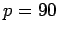cm in front of the lens. What now is the height, location, and nature of the image?

Answer: According to Eq. (367), the image distanceis given by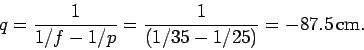Thus, the image is virtual (since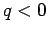), and is located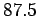cm in front of the lens. According to Eq. (10.24), the magnification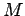of the image is given by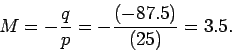Thus, the image is upright (since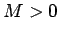), and magnified by a factor of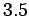. It follows that the height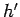of the image is given by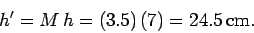If the object is moved such that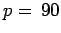cm then the new image distance is given by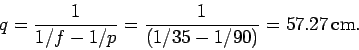Thus, the new image is real (since), and is located 57.27cm behind the lens. The new magnification is given by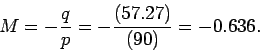Thus, the image is inverted (since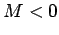), and diminished by a factor of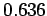. It follows that the new height of the image is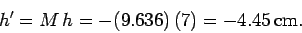Note that the height is negative because the image is inverted.Next: Example 13.4: Diverging lenses Up: Paraxial Optics Previous: Example 13.2: Convex mirrors
Richard Fitzpatrick 2007-07-14